Next: Eigenfunctions of Orbital Angular Up: Orbital Angular Momentum Previous: Eigenvalues of Orbital Angular

# Rotation Operators

Consider a particle whose position is described by the spherical coordinates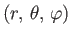. The classical momentum conjugate to the azimuthal angle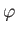is the-component of angular momentum,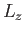. According to Section 2.5, in quantum mechanics, we can always adopt the Schrödinger representation, for which ket space is spanned by the simultaneous eigenkets of the position operators,,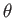, and, andtakes the form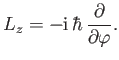(4.57)

Incidentally, it is legitimate to write the previous equation because there is nothing in Section 2.5 that specifies that we have to use Cartesian coordinates--the representation (2.74) works for any well-defined set of coordinates.

Consider an operator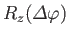that rotates the system through an angle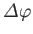about the-axis. This operator is very similar to the operator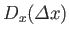, introduced in Section 2.8, which translates the system a distance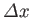along the-axis. We were able to demonstrate in Section 2.8 that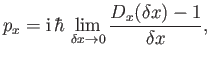(4.58)

where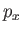is the linear momentum conjugate to. There is nothing in our derivation of this result that specifies thathas to be a Cartesian coordinate. Thus, the result should apply just as well to an angular coordinate. We conclude that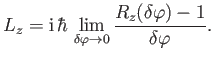(4.59)

According to Equation (4.59), we can write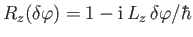(4.60)

in the limit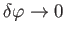. In other words, the angular momentum operatorcan be used to rotate the system about the-axis by an infinitesimal amount. We say thatis the generator of rotation about the-axis. The previous equation implies that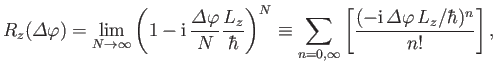(4.61)

which reduces to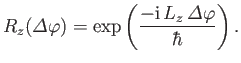(4.62)

Note thathas all of the properties we would expect a rotation operator to possess: that is,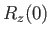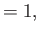(4.63)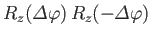(4.64)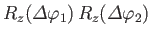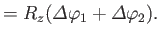(4.65)

(See Exercises 7 and 8.)

Suppose that the system is in a simultaneous eigenstate of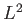and. As before, this state is represented by the eigenket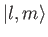, where the eigenvalue ofis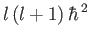, and the eigenvalue ofis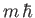. We expect the wavefunction to remain unaltered if we rotate the systemdegrees about the-axis. Thus,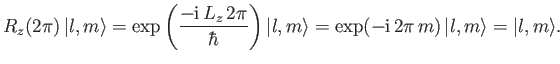(4.66)

We conclude that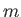must be an integer. This implies, from the previous section, thatmust also be an integer. Thus, an orbital angular momentum can only take integer values of the quantum numbersand.

Consider the action of the rotation operatoron an eigenstate possessing zero angular momentum about the-axis (i.e., anstate). We have(4.67)

Thus, the eigenstate is invariant to rotations about the-axis. Clearly, its wavefunction must be symmetric about the-axis.

There is nothing special about the-axis, so we can write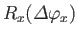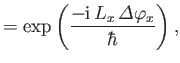(4.68)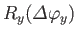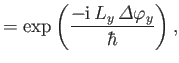(4.69)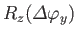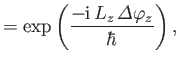(4.70)

by analogy with Equation (4.62). Here,denotes an operator that rotates the system through an angle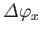about the-axis, et cetera. Suppose that the system is in an eigenstate of zero overall orbital angular momentum (i.e., anstate). We know that the system is also in an eigenstate of zero orbital angular momentum about any particular axis. This follows becauseimplies, according to the previous section, and we can choose the-axis to point in any direction. Thus,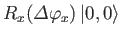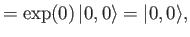(4.71)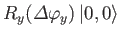(4.72)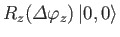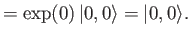(4.73)

Clearly, a zero angular momentum state is invariant to rotations about any axis. Such a state must possess a spherically symmetric wavefunction.

Note that, in general, a rotation about the-axis does not commute with a rotation about the-axis. In other words, if a physical system is rotated through an angleabout the-axis, and then through an angle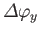about the-axis, it ends up in a different state to that obtained by rotating through an angleabout the-axis, and then through an angleabout the-axis. In quantum mechanics, this implies that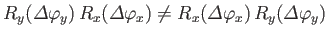, or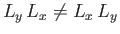. [See Equations (4.68)-(4.70) and Exercise 3.] Thus, the noncommuting nature of the angular momentum operators is a direct consequence of the fact that rotations do not commute.Next: Eigenfunctions of Orbital Angular Up: Orbital Angular Momentum Previous: Eigenvalues of Orbital Angular
Richard Fitzpatrick 2016-01-22